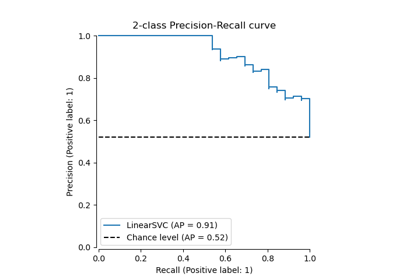# sklearn.preprocessing.label_binarize¶

sklearn.preprocessing.label_binarize(y, *, classes, neg_label=0, pos_label=1, sparse_output=False)[source]

Binarize labels in a one-vs-all fashion.

Several regression and binary classification algorithms are available in scikit-learn. A simple way to extend these algorithms to the multi-class classification case is to use the so-called one-vs-all scheme.

This function makes it possible to compute this transformation for a fixed set of class labels known ahead of time.

Parameters:
yarray-like

Sequence of integer labels or multilabel data to encode.

classesarray-like of shape (n_classes,)

Uniquely holds the label for each class.

neg_labelint, default=0

Value with which negative labels must be encoded.

pos_labelint, default=1

Value with which positive labels must be encoded.

sparse_outputbool, default=False,

Set to true if output binary array is desired in CSR sparse format.

Returns:
Y{ndarray, sparse matrix} of shape (n_samples, n_classes)

Shape will be (n_samples, 1) for binary problems. Sparse matrix will be of CSR format.

LabelBinarizer

Class used to wrap the functionality of label_binarize and allow for fitting to classes independently of the transform operation.

Examples

>>> from sklearn.preprocessing import label_binarize
>>> label_binarize([1, 6], classes=[1, 2, 4, 6])
array([[1, 0, 0, 0],
[0, 0, 0, 1]])


The class ordering is preserved:

>>> label_binarize([1, 6], classes=[1, 6, 4, 2])
array([[1, 0, 0, 0],
[0, 1, 0, 0]])


Binary targets transform to a column vector

>>> label_binarize(['yes', 'no', 'no', 'yes'], classes=['no', 'yes'])
array([,
,
,
])


## Examples using sklearn.preprocessing.label_binarize¶Precision-Recall

Precision-Recall